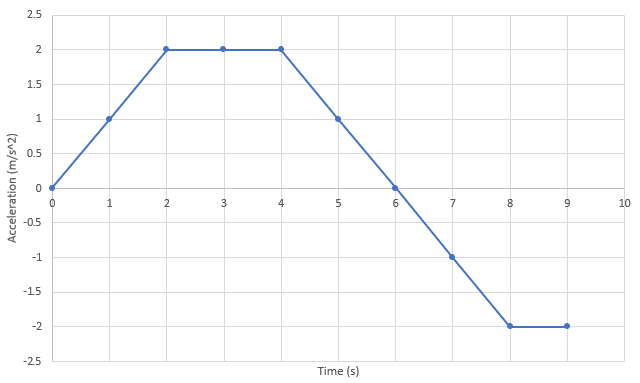## Acceleration Graph

The below graph plots the acceleration of a motorcycle racing on a track. If the bike started from rest, what is its speed at the 7 seconds mark?Hint
Speed is the velocity integral with respect to time:
$$Speed=initial\:speed+\int_{0}^{t}a\:dt$$$Hint 2 By solving an integral, you are calculating the area under the curve. Thus, we can break down the graph by segments: • 0-2 is a triangle • 2-4 is a rectangle • 4-6 is a triangle • 6-7 is a triangle (in the negative direction) Speed is the velocity integral with respect to time: $$Speed=initial\:speed+\int_{0}^{t}a\:dt$$$
$$=0+\int_{0}^{2}a\:dt+\int_{2}^{4}a\:dt+\int_{4}^{6}a\:dt-\int_{6}^{7}a\:dt$$$By solving an integral, you are calculating the area under the curve. Thus, we can break down the graph by segments: • 0-2 is a triangle: $$\frac{1}{2}bh=\frac{1}{2}(2)(2)=2$$ • 2-4 is a rectangle: $$bh=2(2)=4$$ • 4-6 is a triangle: $$\frac{1}{2}bh=\frac{1}{2}(2)(2)=2$$ • 6-7 is a triangle (in the negative direction): $$-\frac{1}{2}bh=-\frac{1}{2}(1)(1)=-\frac{1}{2}$$ Combining all the segments together to find the speed at 7 seconds from initial launch: $$0+2+4+2-\frac{1}{2}=7.5\:m/s$$$
7.5 m/s